# Template Classes in C++

## Template Classes in C++

In this article, I am going to discuss Template Classes in C++. Please read our previous article where we discussed C++ Class and Constructors. The C++ programming language supports generic functions and generic classes. Generic functions are called template functions and generic classes are called template classes.

##### Example:

Let us understand the Generic classes and Functions with an example. First, we will explain the below example. Then we will see what does it mean by generic and how we will convert it into a generic class. Please have a look at the below code. As you can see in the below image, there is a class called Arithmetic with two data members ‘a’ and ‘b’ of integer type. Then there is a constructor which is taking two parameters, again we have taken parameters with the same names ‘a’ and ‘b’. Then we have two simple functions for adding those two numbers and subtracting those two numbers and returning the result.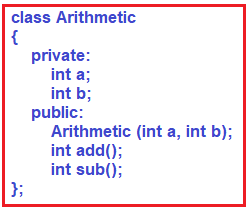So, this is a simple arithmetic class. Now let us focus on the template. Let us implement the three functions (2 member functions and 1 constructor) outside the class using the scope resolution operator as shown below.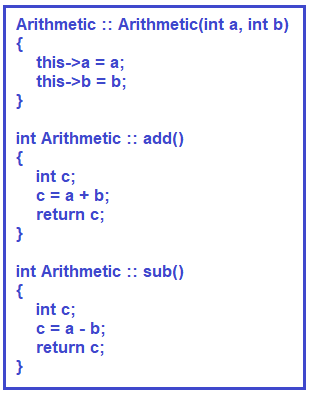If you look at the above image, we have one parameterized constructor which taking two parameters with the same name a and b and initializing the class data members a and b. To differentiate the class members and parameter we have used this object and while initializing we are using the arrow (->) operator. The next two functions i.e. add and sub are straightforward. They simply do the arithmetic addition and subtraction and returning the result. Now let us proceed and understand the Generic.

##### Generic Classes:

Now, let us talk about generic classes. For understanding that first of all, let us look at the Arithmetic class. The arithmetic class is performing arithmetic operations i.e. addition and subtraction on the integer type data. What about float-type data? If we want to use a long integer or if we want to use double, then what? For them, this Arithmetic class will not work. We have to write a separate class for it. We should write a separate class for the floating-point types of data and performing the arithmetic operation.

##### Shall we write two different classes just for a change of data type?

The answer is a big No. C++ says that you can use the same class for multiple data types at a time. You can use only one data type at a time and it works for any type of data. It is called a generic class and that is defined as a template.

Now, let us convert this Arithmetic class into a generic class using a template so that it can operate on different data types and perform arithmetic operations.

##### How to change an existing class into a generic class?

We have written the Arithmetic class. Let us convert this Arithmetic class into generic. For understanding this please have a look at the following code. The left-hand side code is without a generic class and the right-hand side code with a generic class.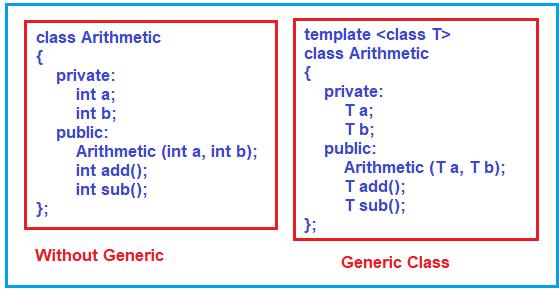As you can see at the top of the class, we need to write the template <class T> to make the class a template class. Here T is the generic type. And wherever you want generic use the type T. It is not mandatory to use T everywhere when you find any data type in your class. As you can see in the Generic class, I have used type T to declare the data members. And in the constructor, I have also used type T for the parameters. Again, the return of the method is also changed to T.

##### Changes in Constructor and Methods:

Now we need to use the same template class in the constructor as well as in methods. For better understanding, please have a look at the following image and observe the changes carefully. Please note in every function and constructor where we want a template, first we need to declare the template. The second change that we did is, wherever we use the Arithmetic class, we need to mention the template type. Again, the final changes we have replaced all the types with T.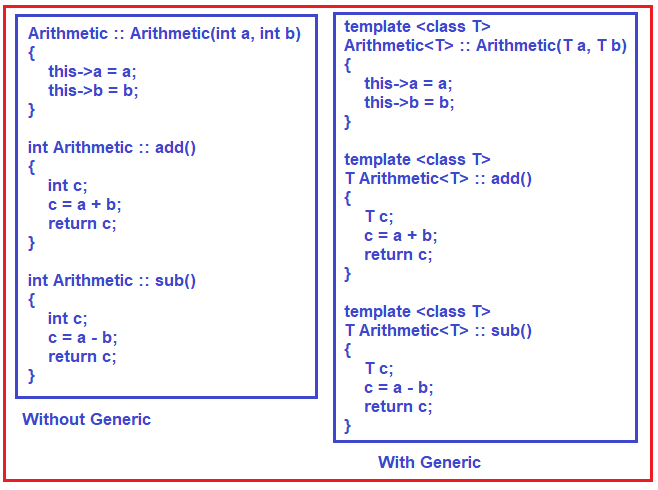Note: In our example, we have replaced all the types with generic type T, but it is not mandatory. So, whenever you are using a template, do the changes carefully.

##### Main Method:

Let us see how to use the template in our main method. For better understanding, please have a look at the below code. First, we are creating an object of Arithmetic. Arithmetic is a template, so while creating the object, we need to mention the data type of the template. In our example, we mention the type as int. So, wherever we used the type T, it will be replaced with the data type int. As the Template is of type int, now while creating the object, we can pass two integer numbers to initialize the data members a and b through the parameterized constructor. Once the object is created then we can call the member functions using the object and dot operator.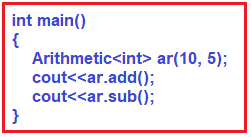Now if you perform arithmetic operations on the Float data type. Then you need to use float while creating the object as shown in the below object.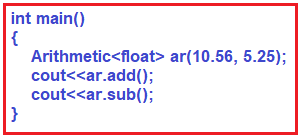##### The complete code is given below.
```#include <iostream>
using namespace std;

template <class T>
class Arithmetic
{
private:
T a;
T b;
public:
Arithmetic (T a, T b);
T sub();
};

template <class T>
Arithmetic<T> :: Arithmetic(T a, T b)
{
this->a = a;
this->b = b;
}

template <class T>
{
T c;
c = a + b;
return c;
}

template <class T>
T Arithmetic<T> :: sub()
{
T c;
c = a - b;
return c;
}

int main()
{
Arithmetic<int> ar1(10, 5);